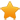Switch Editions?
CancelChannel: CodeSection,代码区,网络安全 - CodeSec
Viewing all articles

# Optimising treehash calculations

0
0

I’ve been using my Glacier Push utility for a while now and it has been working well. But recently I noticed that on really large files the initial memory usage spiked, and then fell off. I suspected that the treehash calculation (implemented in Haskell) was not efficient so I re-implemented it in plain C.

The first thing was to write a utility to calculate the SHA256 of a buffer. Fortunately the OpenSSL docs have lots of examples to work from. Ignoring error handling, this is fairly straightforward:

void sha256(unsigned char *buffer, unsigned int buffer_size, unsigned char *output) { EVP_MD_CTX *mdctx; const EVP_MD *md; unsigned int md_len; md = EVP_get_digestbyname("SHA256"); assert(md != NULL); mdctx = EVP_MD_CTX_new(); EVP_DigestInit_ex(mdctx, md, NULL); EVP_DigestUpdate(mdctx, buffer, buffer_size); EVP_DigestFinal_ex(mdctx, output, &md_len); EVP_MD_CTX_free(mdctx); assert(md_len == DIGEST_SIZE); }

The next thing is the tree hash algorithm . This is basically a Merkle tree on SHA256.

In short, we divide the file into 1Mb blocks and hash each of them. Then we hash pairs, pairs of pairs, and so on, until we have one block left. For example a 4Mb file would take two steps of hashing:

We don’t need a full tree ADT because we have an upper bound on the number of blocks (the file on disk is fixed) so we can make do with a buffer for the hashes and a temp buffer for writing intermediate results. The algorithm boils down to this loop (full source is here ):

while (nr_blocks_left > 1) { int pairs_to_process = nr_blocks_left/2; // sha256 the pairs for(unsigned int k = 0; k < pairs_to_process; k++) sha256(&digests[2*k*DIGEST_SIZE], 2*DIGEST_SIZE, &next_tmp[k*DIGEST_SIZE]); // Copy back into digests. for(int i = 0; i < pairs_to_process*DIGEST_SIZE; i++) digests[i] = next_tmp[i]; // If there is a block left over, copy it. if (nr_blocks_left % 2 == 1) { for(int i = 0; i < DIGEST_SIZE; i++) digests[pairs_to_process*DIGEST_SIZE + i] = digests[(nr_blocks_left-1)*DIGEST_SIZE + i]; } if (nr_blocks_left % 2 == 0) nr_blocks_left = pairs_to_process; else nr_blocks_left = pairs_to_process + 1; }

Our C interface to treehash has this declaration:

char * treehash(char *fname, unsigned long long start, unsigned long long end);

To call this from Haskell we do some FFI:

{-# LANGUAGE ForeignFunctionInterface #-} module TreehashFFI (treehash_FFI, treehash_FFI') where import System.Posix.Files import Foreign import Foreign.C.Types import Foreign.C.String foreign import ccall "treehash.h treehash" c_treehash :: CString -> CULong -> CULong -> IO CString treehash_FFI :: String -> Int64 -> Int64 -> IO String treehash_FFI filename start end = withCString filename \$ \c_filename -> do ptrHash <- c_treehash c_filename (fromIntegral start) (fromIntegral end) h <- peekCString ptrHash free ptrHash return h

And we can write a nice wrapper in Haskell by providing the file size:

treehash_FFI' :: String -> IO String treehash_FFI' fp = do stat <- getFileStatus fp let lastByte = fromIntegral \$ toInteger (fileSize stat) - 1 treehash_FFI fp 0 lastByte

To monitor the memory performance I logged the output of ps and plotted the results using gnuplot (scripts borrowed from Bruno Girin ).

Memory usage (as percentage of 16Gb total memory) when pushing a 7.5Gb file to Glacier:

The C version uses so little memory that it barely shows up on the plot. Both implementations use about the same amount of memory after the treehash calculation.

Viewing all articles

More Pages to Explore .....